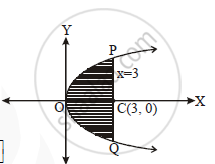# Find the Area of the Region Bounded by the Parabola y^2 = 16x and the Line x = 3. - Mathematics and Statistics

Find the area of the region bounded by the parabola y2 = 16x and the line x = 3.

#### Solution

y^2 = 16x

area =2int_0^3ydx

=2int_0^34 sqrtxdx

=8 2/3[x^(3/2)]_0^3

=16/3[3^(3/2)]

=16/3[3xx3^(1/2)]

=16[3^(1/2)]

=16sqrt3 sq.unitsConcept: Area of the Region Bounded by a Curve and a Line
Is there an error in this question or solution?